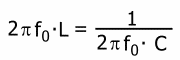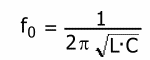# What is resonance in AC circuits

Start | Basics | AC technology | Telecommunications | Digital technology | Tables | Test tasks | Quiz | PDF files

display

Resonant circuit

If you connect a coil (inductance) and a capacitor (capacitance) together, a Resonant circuit. The interconnection can take place either in the form of a series connection or in the form of a parallel connection.
If a voltage is briefly applied to such a circuit, the capacitor charges up to the maximum voltage value of the voltage source. If you then switch off the voltage again, the capacitor discharges again through the coil. Through the coil, the capacitor charges again with the opposite polarity. This process is repeated several times, with the voltage level becoming lower and lower due to the effective resistances in the resonant circuit. In this way a damped oscillation.
A special case occurs when the inductive and capacitive reactance are equal and thus cancel each other out. In this case, the resonant circuit only has an effective resistance. This case is known as resonance.
The frequency at which this occurs is called Resonance frequency f0 designated.

XL. = XC.

In the case of resonance, the inductive reactance is XL. and the capacitive reactance XC. same size.one sets for XL. and XC. the corresponding equations and then solves this equation according to f0 on so you get:Resonance frequency of the oscillating circuit
Test task for the resonance frequency

display

Our book tips on electrical engineering
Imprint | privacy